International
Tables for
Crystallography
Volume D
Physical properties of crystals
Edited by A. Authier

International Tables for Crystallography (2006). Vol. D, ch. 1.4, pp. 100-101

## Section 1.4.2. Grüneisen relation

H. Küppersa*

aInstitut für Geowissenshaften, Universität Kiel, Olshausenstrasse 40, D-24098 Kiel, Germany
Correspondence e-mail: kueppers@min.uni-kiel.de

### 1.4.2. Grüneisen relation

| top | pdf |

Thermal expansion of a solid is a consequence of the anharmonicity of inter­atomic forces (see also Section 2.1.2.8). If the potentials were harmonic, the atoms would oscillate (even with large amplitudes) symmetrically about their equilibrium positions and their mean central position would remain unchanged. In order to describe thermal expansion, the anharmonicity is most conveniently accounted for by means of the so-called quasiharmonic approximation', assuming the lattice vibration frequencies ω to be independent of temperature but dependent on volume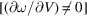. Anharmonicity is taken into account by letting the crystal expand, but it is assumed that the atoms vibrate about their new equilibrium positions harmonically, i.e. lattice dynamics are still treated in the harmonic approximation. The assumption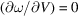, which is made for the harmonic oscillator, is a generalization of the postulate that the frequency of a harmonic oscillator does not depend on the amplitude of vibration.

This approach leads, as demonstrated below, to the Grüneisen relation, which combines thermal expansion with other material constants and, additionally, gives an approximate description of the temperature dependence of thermal expansion (cf. Krishnan et al., 1979; Barron, 1998).

For isotropic media, the volume expansion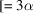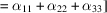, cf. (1.4.1.2), can be expressed by the thermodynamic relation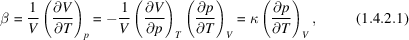κ being the isothermal compressibility. To obtain the quantity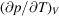, the pressure p is deduced from the free energy F, whose differential is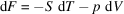, i.e. from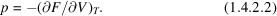In a crystal consisting of N unit cells with p atoms in each unit cell, there are 3p normal modes with frequencies(denoted by an index s running from 1 to 3p) and with N allowed wavevectors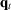(denoted by an index t running from 1 to N). Each normal mode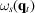contributes to the free energy by the amount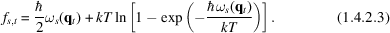The total free energy amounts, therefore, to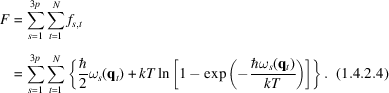From (1.4.2.2)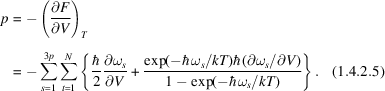The last term can be written as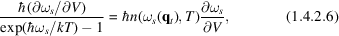where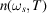is the Bose–Einstein distribution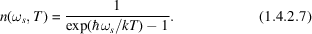Differentiation of (1.4.2.5)and (1.4.2.6)with respect to temperature at constant volume [see (1.4.2.1)] yields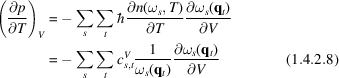with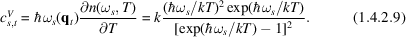This quantity,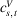(the Einstein function), is the well known contribution of the normal modeto the specific heat (at constant volume):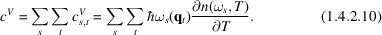Equation (1.4.2.8)can be simplified by the introduction of an individual Grüneisen parameter'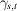for each normal mode: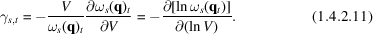Equation (1.4.2.8)then reads [with (1.4.2.1)]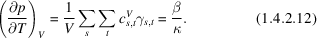Based on these individual parameters, an average (or overall mode-independent) Grüneisen parameter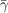can be defined as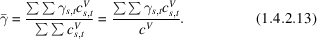In this averaging process, the contribution of each normal mode tois weighted in the same way as it contributes to the specific heat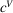[see (1.4.2.10)]. Equations (1.4.2.12)and (1.4.2.13)lead to the Grüneisen relation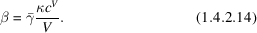The above derivation was made for isotropic media. For anisotropic media,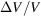is replaced by the strain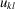and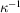is replaced by the stiffness tensor[cf. Chapter 2.1and equation (2.1.2.75)]. Then the Grüneisen parameter turns out to be a second-rank tensor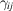: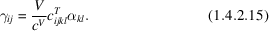In the Debye approximation, the mode frequencies scale linearly with the cut-off frequency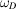. Therefore, with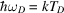, the average isotropic Grüneisen parameter is calculated to be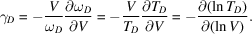Since, in the Debye theory,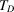is independent of temperature,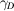turns out to be independent of temperature. As κ and V are only weakly temperature dependent, the thermal expansion β should then, according to (1.4.2.14), roughly behave like, i.e. β should be proportional toat very low temperatures, and should be approximately constant for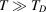(the Dulong–Petit law). This behaviour is found to be approximately satisfied for many compounds, even with different types of interatomic interaction, and γ takes values roughly between 1 and 2. Even in the case of crystals with highly anisotropic elastic and thermal behaviour, the three principal values of the tensor[(1.4.2.15)] are comparably uniform, having values of about 2 (Küppers, 1974).

Effectively, γ shows a certain more or less pronounced dependence on temperature. The individualare assumed to be temperature independent. However, being an average over the whole spectrum of excited modes [cf. (1.4.2.13)],will not necessarily have the same value at low temperatures (when only low frequencies are excited) as at high temperatures (when all modes are excited). Two limiting cases can be considered:

 (1) At very high temperatures, all normal modes contribute by an equal amount and the overallbecomes simply the mean value of all.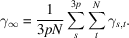(2) At very low temperatures, only the lower frequencies contribute. If only the acoustic branches are considered,can be related to the velocities of elastic waves. In the long-wavelength limit, dispersion is neglected, i.e.is proportional to ω: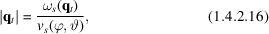where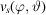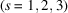describes the velocities of the three elastic waves propagating in a direction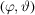. The density of vibrational states for each acoustic branch in reciprocal space increases with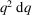. From (1.4.2.16), it follows that the number of normal modes in an increment of solid angle in q space,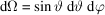, within a frequency interval ω to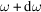, is proportional to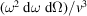. The summation over t can be converted into an integration over ω and Ω, leading to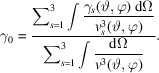Thecan be calculated if the elastic constants are known. For isotropic solids, the term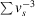can be replaced (as done in Debye's theory of heat capacity) by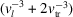, with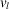being the velocity of the longitudinal wave and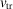the velocity of the transverse waves.

In metals, the conduction electrons and magnetic interactions yield contributions to the free energy and to the specific heat. Accordingly, expression (1.4.2.14)can be augmented by introduction of an electronic Grüneisen parameter',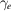, and a magnetic Grüneisen parameter',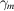, in addition to the `lattice Grüneisen parameter',, considered so far: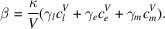### References

Barron, T. H. K. (1998). Generalized theory of thermal expansion of solids. In Thermal expansion of solids, edited by C. Y. Ho, ch. 1. Materials Park, Ohio: ASM International.
Krishnan, R. S., Srinivasan, R. & Devanarayanan, S. (1979). Thermal expansion of solids. Oxford: Pergamon.
Küppers, H. (1974). Anisotropy of thermal expansion of ammonium and potassium oxalates. Z. Kristallogr. 140, 393–398.